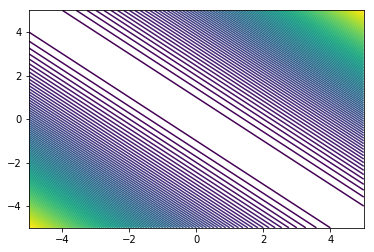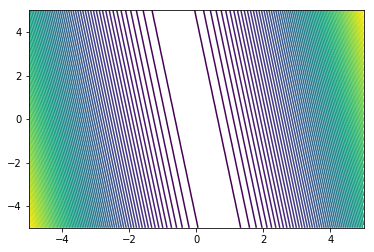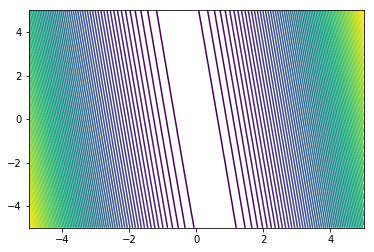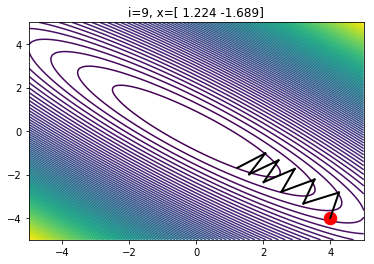In :
import autograd.numpy as np
import matplotlib.pyplot as plt
%matplotlib inline
np.set_printoptions(precision=3)
import time


This tutorial explores optimization for machine learning.

We will implement a function and then use gradient-based optimization to find it's minimum value. For ease of visualization we will consider scalar functions of 2-d vectors. In other words $f: \mathbb{R}^2 \rightarrow \mathbb{R}$

One example of such a function is the simple quadratic $$f(x) = \frac{1}{2} x^T x = \frac{1}{2}(x_0^2 + x_1^2)$$ where $x_i$ represents the $i$-th entry of the vector $x$.

Let's implement this in code and print $f(x)$ for a few random $x$

In :
def f(x):
return 0.5*np.dot(x.T, x)

for _ in range(5):
x = np.random.randn(2)  # random 2-d vector
print('x={}, f(x)={:.3f}'.format(x, f(x)))

x=[-0.826 -1.409], f(x)=1.333
x=[0.491 0.28 ], f(x)=0.159
x=[0.9   0.487], f(x)=0.524
x=[-0.258  0.969], f(x)=0.503
x=[ 0.139 -1.164], f(x)=0.687


This simple function has minimum value $f(x^*)=0$ given by $x^* = [0, 0]^T$.

Let's look at the more general quadratic $$f(x) = \frac{1}{2}x^T A x$$ where $A \in \mathbb{R}^{2 \times 2}$ is a positive semi-definite matrix.

Notice that we get the previous function when we set $A$ to the identity matrix $I$.

We can think of this function as a quadratic bowl whose curvature is specified by the value of $A$. This is evident in the isocontour plots of $f(x)$ for various $A$. Let's take a look.

In :
# helper function yielding a random positive semi-definite matrix
def random_psd_matrix(seed=None):
"""return random positive semi-definite matrix with norm one"""
np.random.seed(seed)
A = np.random.randn(2,2)
A = np.dot(A.T,A)
A = np.dot(A.T,A)
A = A / np.linalg.norm(A)
return A

# define forward function
def f(x, a):
"""f(x) = x^T A x"""
y = 0.5*np.dot(x.T, np.dot(a, x))
return y

# helper function for isocontour plotting
def plot_isocontours(one_d_grid, g):
"""
first makes a 2d grid from the 1d grid
then plots isocontours using the function g
"""
X,Y = np.meshgrid(one_d_grid, one_d_grid)  # build 2d grid
Z = np.zeros_like(X)
# numpy bonus exercise: can you think of a way to vectorize the following for-loop?
for i in range(len(X)):
for j in range(len(X.T)):
Z[i, j] = g(np.array((X[i, j], Y[i, j])))  # compute function values
fig, ax = plt.subplots()
ax.contour(X, Y, Z, 100)
return fig, ax

In :
for A in [np.ones((2, 2)), random_psd_matrix(), random_psd_matrix()]:  # try a few values of A
print('A={}'.format(A))
fig, ax = plot_isocontours(np.linspace(-5, 5, 100), lambda x: f(x, A))
plt.show()
time.sleep(1.0)

A=[[1. 1.]
[1. 1.]]A=[[0.981 0.135]
[0.135 0.019]]A=[[0.988 0.111]
[0.111 0.013]]Now let's learn how to find the minimal value of $f(x)$. We need to solve the following optimization problem: $$x^* = \underset{x}{\arg \min} \quad \frac{1}{2}x^T A x = \underset{x}{\arg \min} \quad f(x)$$

Modern machine learning optimization tools rely on gradients. Consider gradient descent, which initializes $x_0$ at random then follows the update rule $$x_{t+1} \leftarrow x_t - \eta \nabla_x f(x_t)$$ where $\eta$ represents the learning rate.

So we need to compute $\nabla_x f(x)$. For simple functions like ours, we can compute this analytically: $$\nabla_x f(x) = \nabla_x \left( \frac{1}{2}x^T A x \right) = A x$$

In other words, to compute the gradient of $f(x)$ at a particular $x=x'$, we matrix multiply $A$ with $x'$

But deriving the analytic gradients by hand becomes painful as $f$ gets more complicated. Instead we can use automatic differentation packages like autograd to do this hard work for us. All we need to do is specify the forward function.

Let's take a look at the two approaches:

In :
# define df/dx via automatic differentiation
# ^ the second argument of grad specifies which argument we're differentiating with respect to

# define df/dx analytically
return np.dot(A, x)

for A in [np.zeros((2, 2)), np.eye(2), random_psd_matrix()]:  # try a few values of A
x = np.random.randn(2)  # generate x randomly
print('')
print('x={}\nA={}\nf(x,A)={:.3f}\ndf/dx={}'.format(x, A, f(x,A), df_dx(x,A)))
assert np.isclose(np.sum((df_dx(x, A) - analytic_gradient(x, A)))**2, 0.), 'bad maths'  # unit test


x=[ 0.016 -1.096]
A=[[0. 0.]
[0. 0.]]
f(x,A)=0.000
df/dx=[0. 0.]

x=[ 0.058 -1.24 ]
A=[[1. 0.]
[0. 1.]]
f(x,A)=0.770
df/dx=[ 0.058 -1.24 ]

x=[0.816 0.82 ]
A=[[ 0.597 -0.491]
[-0.491  0.403]]
f(x,A)=0.006
df/dx=[ 0.085 -0.069]


Now that we know how to compute $\nabla_x f(x)$ using autograd, let's implement gradient descent.

To make the implementation of GD crystal clear, let's break this update expression from above into two lines: $$\delta_{t+1} \leftarrow - \eta \nabla_x f(x_t)$$ $$x_{t+1} \leftarrow x_t + \delta_{t+1}$$

This yields the following autograd implementation:

In :
A = random_psd_matrix(0)  # argument specifies the random seed
fig, ax = plot_isocontours(np.linspace(-5, 5, 100), lambda x: f(x, A))  # plot function isocontours

# hyperparameters
LEARNING_RATE = 2.0
INITIAL_VAL = np.array([4., -4.])  # initialize

x = np.copy(INITIAL_VAL)
ax.plot(*x, marker='.', color='r', ms=25)  # plot initial values

from IPython import display

for i in range(10):
#    input("Press Enter to continue...")  # optional; Enter key triggers next update
#    time.sleep(0.5)  # optional; slow down animation if the flickering is distracting

x_old = np.copy(x)
delta = -LEARNING_RATE*df_dx(x, A)  # compute gradient times learning rate
x += delta  # update params

# plot
ax.plot([x_old, x], [x_old, x], linestyle='-', color='k',lw=2)  # plot a line connecting old and new param values
fig.canvas.draw()
ax.set_title('i={}, x={}'.format(i, x))
display.display(fig)
display.clear_output(wait=True)

print('final value f(x)={:.3f} at x={}'.format(f(x, A), x))

final value f(x)=0.236 at x=[ 1.224 -1.689]Cool! Now let's try gradient descent with momentum. The hyperparameters are the learning rate $\eta$ and momentum value $\alpha \in [0, 1)$.

We randomly initialize $x_0$ like before. We initialize $\delta_0$ to the zero vector. Then proceed with updates as: $$\delta_{t+1} \leftarrow -\eta \nabla_x f(x) + \alpha \delta_t$$ $$x_{t+1} \leftarrow x_t + \delta_{t+1}$$

This yields the following autograd implementation:

In :
fig, ax = plot_isocontours(np.linspace(-5, 5, 100), lambda x: f(x, A))  # plot function isocontours

# initialize
x = np.copy(INITIAL_VAL)
delta = np.zeros(2)
ax.plot(*x, marker='.', color='r', ms=25)  # plot initial values

# hyperparameters
LEARNING_RATE = .1
ALPHA = 0.5

from IPython import display

for i in range(100):
#    input("Press Enter to continue...")  # optional; Enter key triggers next update
#    time.sleep(0.5)  # optional; slow down animation if the flickering is distracting

x_old = np.copy(x)
g = df_dx(x, A)  # compute standard gradient
delta = -LEARNING_RATE*g + ALPHA*delta  # update momentum term
x += delta  # update params

# plot
ax.plot([x_old, x], [x_old, x],'-k',lw=2)  # plot a line connecting old and new param values
fig.canvas.draw()
ax.set_title('i={}, x={}'.format(i, x))
display.display(fig)
display.clear_output(wait=True)

print('final value f(x)={:.3f} at x={}'.format(f(x, A), x))

final value f(x)=0.109 at x=[ 1.631 -1.348]